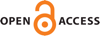Download this articleFor screen For printingRecent IssuesThe Journal About the Journal Editorial Board Editors’ Interests Subscriptions Submission Guidelines Submission Form Policies for Authors Ethics Statement ISSN: 1948-206X (e-only) ISSN: 2157-5045 (print) Author Index To Appear Other MSP JournalsThe strong topology of $\omega$-plurisubharmonic functions

### Antonio Trusiani

Vol. 16 (2023), No. 2, 367–405##### Abstract

On a compact Kähler manifold $\left(X,\omega \right)$, given a model-type envelope $\psi \in \mathrm{PSH}\left(X,\omega \right)$ (i.e., a singularity type) we prove that the Monge–Ampère operator is a homeomorphism between the set of $\psi$-relative finite energy potentials and the set of $\psi$-relative finite energy measures endowed with their strong topologies given as the coarsest refinements of the weak topologies such that the relative energies become continuous. Moreover, given a totally ordered family $\mathsc{𝒜}$ of model-type envelopes with positive total mass representing different singularity types, the sets ${X}_{\mathsc{𝒜}}$ and ${Y}_{\mathsc{𝒜}}$, given as the union of all $\psi$-relative finite energy potentials and of all $\psi$-relative finite energy measures with varying $\psi \in \overline{\mathsc{𝒜}}$, respectively, have two natural strong topologies which extend the strong topologies on each component of the unions. We show that the Monge–Ampère operator produces a homeomorphism between ${X}_{\mathsc{𝒜}}$ and ${Y}_{\mathsc{𝒜}}$.

As an application we also prove the strong stability of a sequence of solutions of complex Monge–Ampère equations when the measures have uniformly ${L}^{p}$-bounded densities for $p>1$ and the prescribed singularities are totally ordered.

##### Keywords
complex Monge-Ampère equations, compact Kähler manifolds, quasi-psh functions
##### Mathematical Subject Classification
Primary: 32W20
Secondary: 32Q15, 32U05
##### Milestones